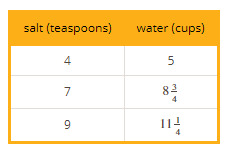PSABPW52 - Cool-Down---Acc7.5 Lesson 3 Comparing Proportional Relationships (8.EE.B.5)
Part A)

Here are recipes for two mixtures of salt and water that taste different.

Information about Salt Mixture A is shown in the table.Salt Mixture B is defined by the equation y = 2.5x, where x is the number of teaspoons of salt and y is the number of cups of water.

If you used 10 cups of water, which mixture would use more salt?

Select one:
Part B)

How much more?

Type your answer below as a number (example: 5, 3.1, 4 1/2, or 3/2):
Part C)